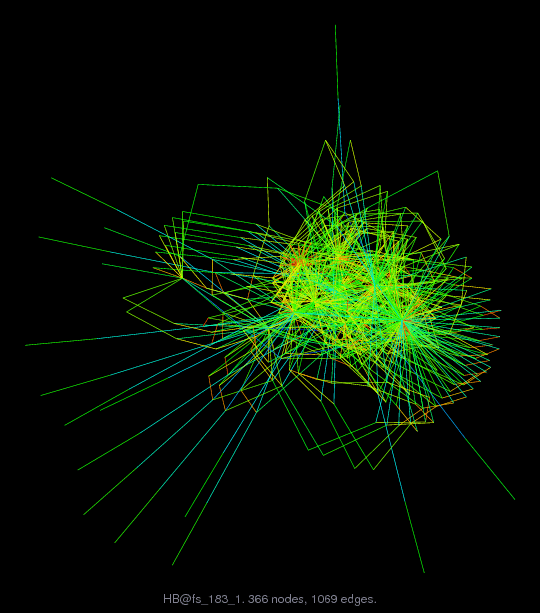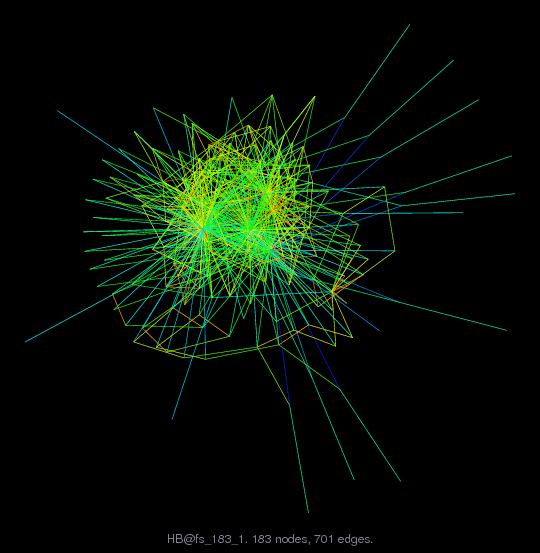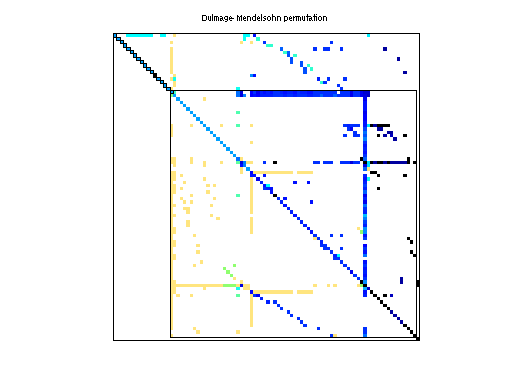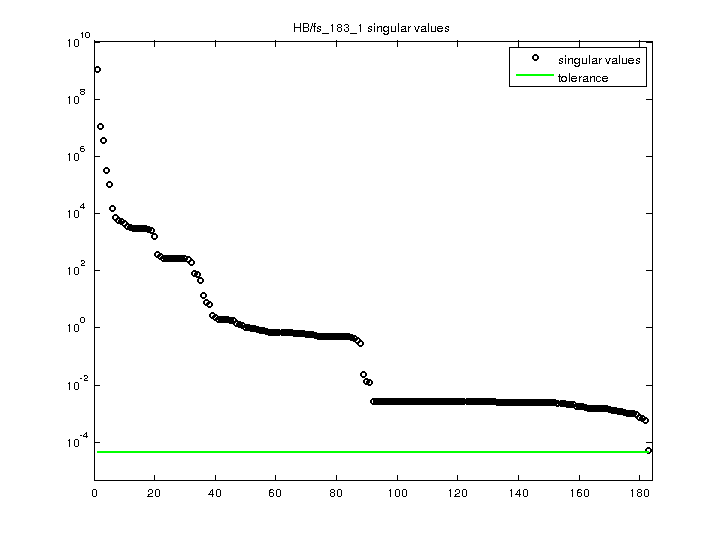Matrix: HB/fs_183_1

Description: UNSYMMETRIC FACSIMILE CONVERGENCE MATRIX(bipartite graph drawing) (graph drawing of A+A')• Matrix group: HB
• download as a MATLAB mat-file, file size: 8 KB. Use UFget(141) or UFget('HB/fs_183_1') in MATLAB.

 Matrix properties number of rows 183 number of columns 183 nonzeros 998 structural full rank? yes structural rank 183 # of blocks from dmperm 37 # strongly connected comp. 37 explicit zero entries 71 nonzero pattern symmetry 42% numeric value symmetry 0% type real structure unsymmetric Cholesky candidate? no positive definite? no

 author A. Curtis editor I. Duff, R. Grimes, J. Lewis date 1983 kind 2D/3D problem sequence 2D/3D problem? yes

Notes:

```next: HB/fs_183_3 first: HB/fs_183_1
```

 Ordering statistics: result nnz(chol(P*(A+A'+s*I)*P')) with AMD 1,064 Cholesky flop count 7.8e+03 nnz(L+U), no partial pivoting, with AMD 1,945 nnz(V) for QR, upper bound nnz(L) for LU, with COLAMD 3,749 nnz(R) for QR, upper bound nnz(U) for LU, with COLAMD 7,285

Note that all matrix statistics (except nonzero pattern symmetry) exclude the 71 explicit zero entries.

 SVD-based statistics: norm(A) 1.12935e+09 min(svd(A)) 5.14889e-05 cond(A) 2.19339e+13 rank(A) 183 sprank(A)-rank(A) 0 null space dimension 0 full numerical rank? yes

 singular values (MAT file): click here SVD method used: s = svd (full (A)) ; status: ok